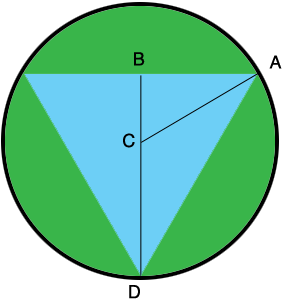SEARCH HOMEMath Central Quandaries & QueriesQuestion from Casey: I have an equilateral triangle inscribed in a circle - this triangle has been bisected to give me 2 right triangles. I know the length of the line bisecting the equilateral triangle is 36 inches. How do I figure out the circumference of the circle and the length of the sides of the equilateral triangle?Hi Casey,

The key to this problem is that in any triangle with angles that measure 30, 60 and 90 degrees the lengths of the sides are in the ratio of $1: 2: \sqrt{3}.$

I drew a diagram of with the center of the circle at $C$ and added a line segment from $C$ to $A,$ one of the vertices of the triangle.Can you complete the problem now?

Write back if you need more assistance,
PennyMath Central is supported by the University of Regina and the Imperial Oil Foundation.